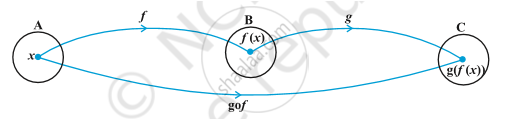HSC Arts 11thMaharashtra State Board
Share

# Composition of Functions and Invertible Function

#### notes

In order to understant the composition of functions we have taken three sets in front of us name them as A,B and C.now if we take a function f which is moving from A to B and then beginning with the stage set B, I take another function g from B to C. If we take a random element , after applying the funtion f it becomes f(x). Now beginning with f(x), this act as the element for the new function g and gives us the final product as g(f(x)), in short the new function which moves from A to C is defined by gof.
So if to define the function we say if
f: A→B & g: B→C, then
gof: A→C defined by gof(x)= g(f(x)) ∀x∈A
Notes:
1) f: A→B and g: B→C then,
range of f should be the starting point of the domain for the next function g for gof to exist. Likewise for fog to exist, the range of g should be subset of domain of f.
2) In general fog ≠ gof
Example- Let f: R→R
"f"(x)= x^2
g: R→R
g(x)= 2x+1
find fog & gof.
Solution- fog(x)= f(g(x))
fog(x)= f(2x+1)= (2x+1)^2
gof(x)= g(f(x))
gof(x)= g(x^2)= 2x^2+1
Invertible functions- Invertible means the function will be one one or onto both, that means the function will be bijective.
This means if f: A→B, then there exists "f"^-1:"B"→"A"
So, if this is was f(x)= y then x= "f"^-1(y)
Example1- If A= {1,2,3}, B= {4,5,6,7} and f={(1,4), (2,5), (3,6)} is a function from A to B. Is it one- one function?
Yes, because every element in set A have different image.
Example2- If f is invertible which is defined as "f"(x)= (3x-4)/5 then write "f"^-1 (x).
Solution- f(x)=y

(3x-4)/5 = y

3x-4 = 5y

x= (5y+4)/3 = "f"^-1 (y)
Theorem 1: If f : X → Y, g : Y → Z and h : Z → S are functions, then ho(gof) = (hog)of.
Proof : We have
ho(gof) (x) = h(gof(x)) = h(g(f(x))), ∀x in X
and (hog) of (x) = hog(f (x)) = h(g(f(x))), ∀x in X.
Hence, ho(gof) = (hog)of.
Theorem 2: Let f : X → Y and g : Y → Z be two invertible functions. Then gof  is also invertible with (gof)^(–1) = f^(–1)og^(–1).
Proof:  To show that gof is invertible with (gof)^(–1) = f^(–1)og^(–1),

it is enough to show that (f^(–1)og^(–1))o(gof) = "I"_X and (gof)o(f^(–1)og^(–1)) = "I"_Z.

Now, (f^(–1)og^(–1))o(gof) = ((f^(–1)og^(–1)) og) of,  byTheorem 1

= (f^(–1)o(g^(–1)og)) of, by Theorem 1

= (f^(–1) oI_Y) of, by definition of g^–1

= "I"_X.

Similarly, it can be shown that (gof)o(f ^(–1) og ^(–1)) = "I"_Z.

### Shaalaa.com

Relations and Functions part 26 (Composition of Functions) [00:10:58]
S
1
0%

S